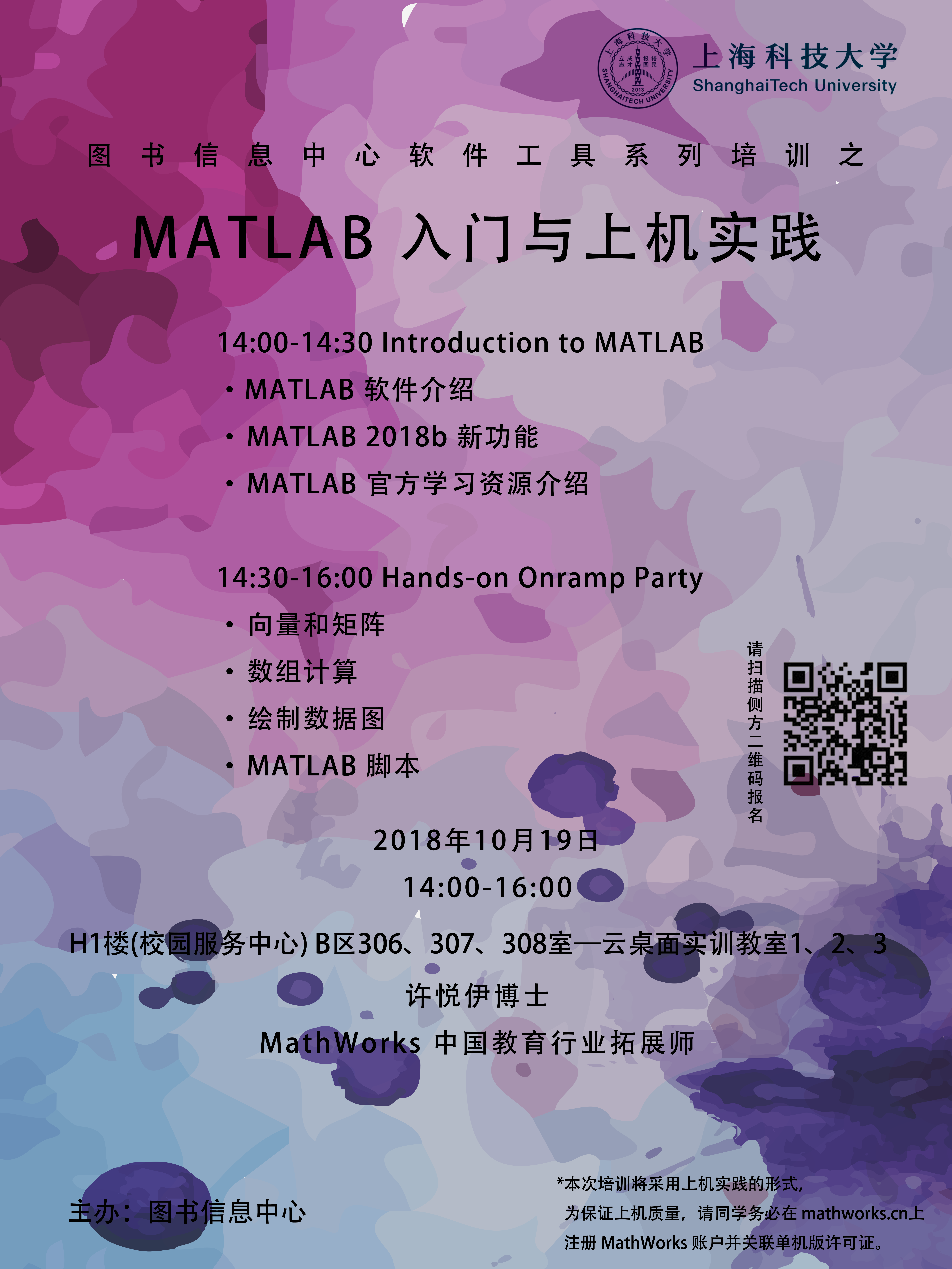# [培训]MATLAB 入门与上机实践

*目前MATLAB 入门与上机实践培训人数已满。为了更好的满足大家的需求，我们在教学中心101增开了远程分会场同步直播，没报上的同学届时可提前安装软件并自带电脑直接到分会场参加培训，请大家互相转告!

*报名链接：https://www.wjx.top/jq/28561387.aspx （限定席位100人，报满即止！）

**注意事项**

1. 为保证上机质量，请同学务必 mathworks.cn上注册 MathWorks 账户并关联单机版许可证。

2. 为保证大家能够学以致用，温故知新，推荐在个人电脑上安装 MATLAB 单机版，下载地址为：http://software.lib.shanghaitech.edu.cn/MatLab/

14:00-14:30 Introduction to MATLAB

• MATLAB 软件介绍

• MATLAB 2018b 新功能

• MATLAB 官方学习资源介绍

14:30-16:00 Hands-on Onramp Party

• 向量和矩阵

• 数组计算

• 绘制数据图

• MATLAB 脚本